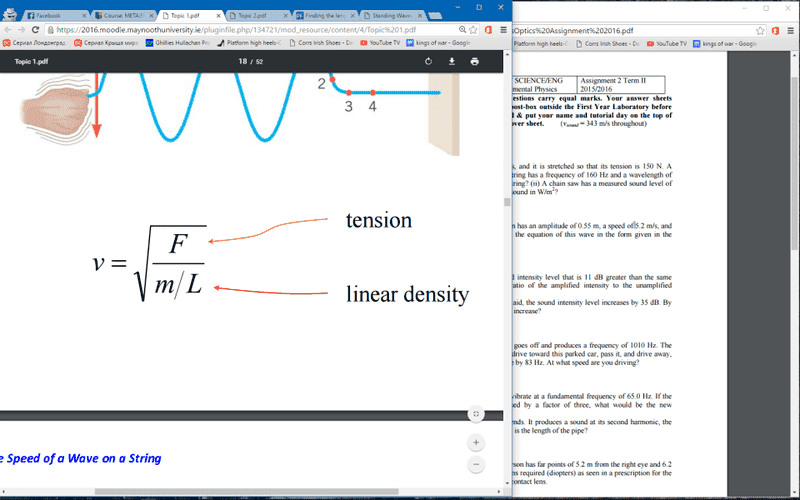# Finding the length of a string given f, F, λ and m

Going through my physics assignment tonight, so that's 10 questions I'll be posting. Since I'm thoroughly hopeless at this subject most of my attempts will be based on my elite google-fu or trawling through lecture notes, bear with me and thanks!

1. Homework Statement

frequency (f)=160hz
Force (F)=150 newtons
wavelength (λ)=0.40m
mass(m)=6 grams

find the length of a string with these properties

## Homework Equations

f(λ)=sqrt [F/(m/L)]

found this formula and the statement "solve for L" for this exact question online.
I put the values in above, I believe I interpreted them right.

## The Attempt at a Solution

It seemed a bit dodge to re-arrange, but i got L on it's own to be:
L= [F/(fλ ^2)]/m
(with values)
[150/(160x0.40)^2]/6=0.0061m...I have a feeling I may be off here XD.

Last edited by a moderator:

## Answers and Replies

berkeman
Mentor
f(λ)=sqrt [F/(m/L)]
What is that equation? The right hand side (RHS) looks like the velocity of waves on a string...

I'm not sure, someone asked the same question as me on some website and that formula along with "solve for L" was the one-sentence reply. I used it because it seems to involve all the values listed in the question and can be used to find L. Is it incorrect?

Edit: see new post

Wait I found a simpler formula based on what you said:I can get v since the wavelength and frequency is given (wavelength=v/frequency).

So if I re-arrange it to get L on its own, then L=[(m)(V^2)]/F, correct?

Last edited:
•berkeman